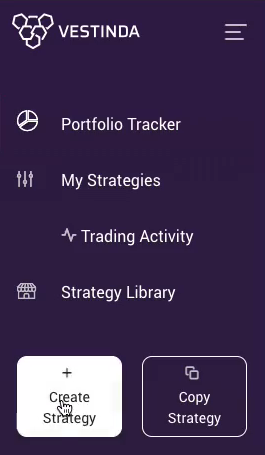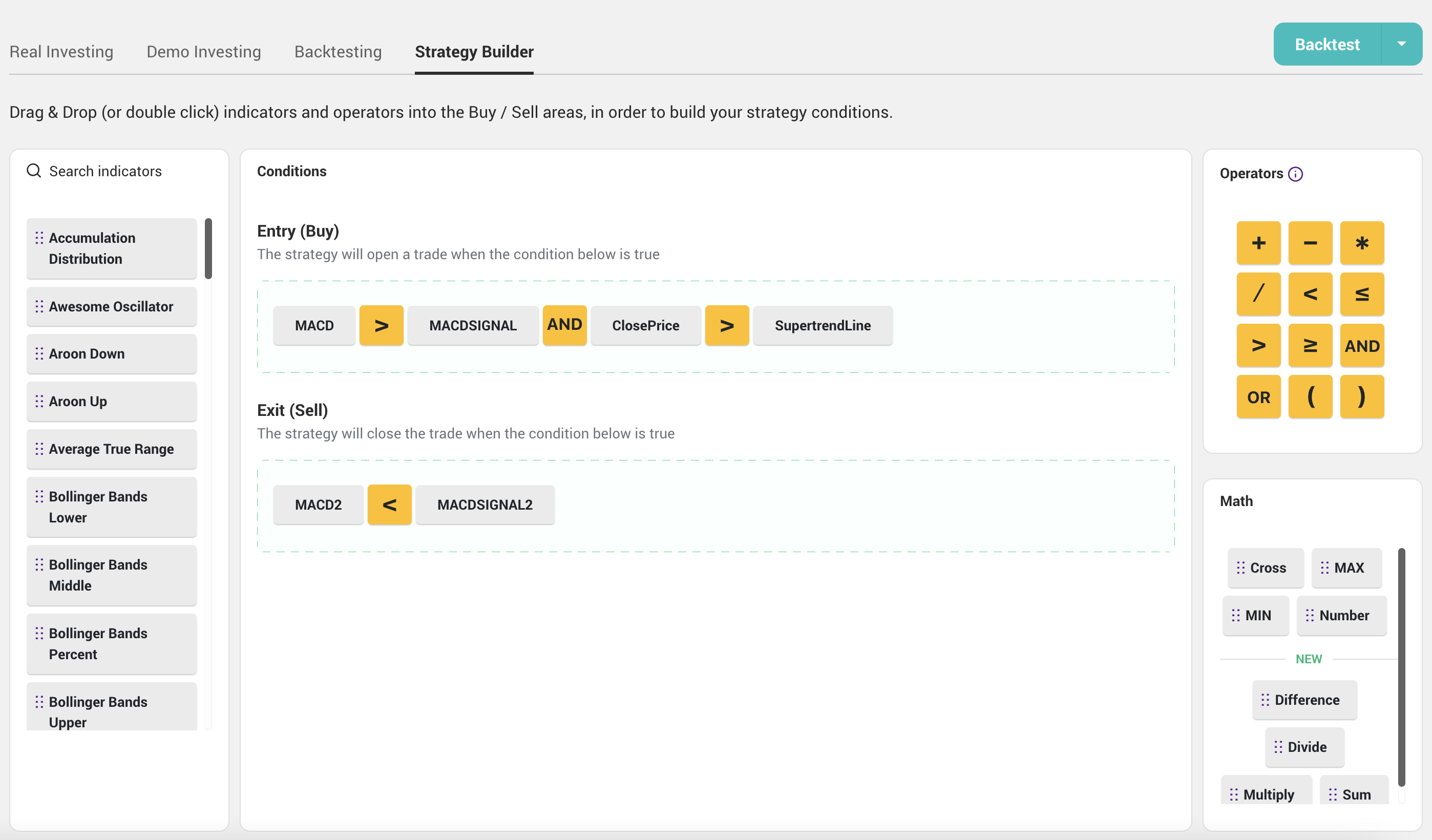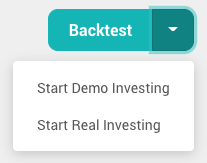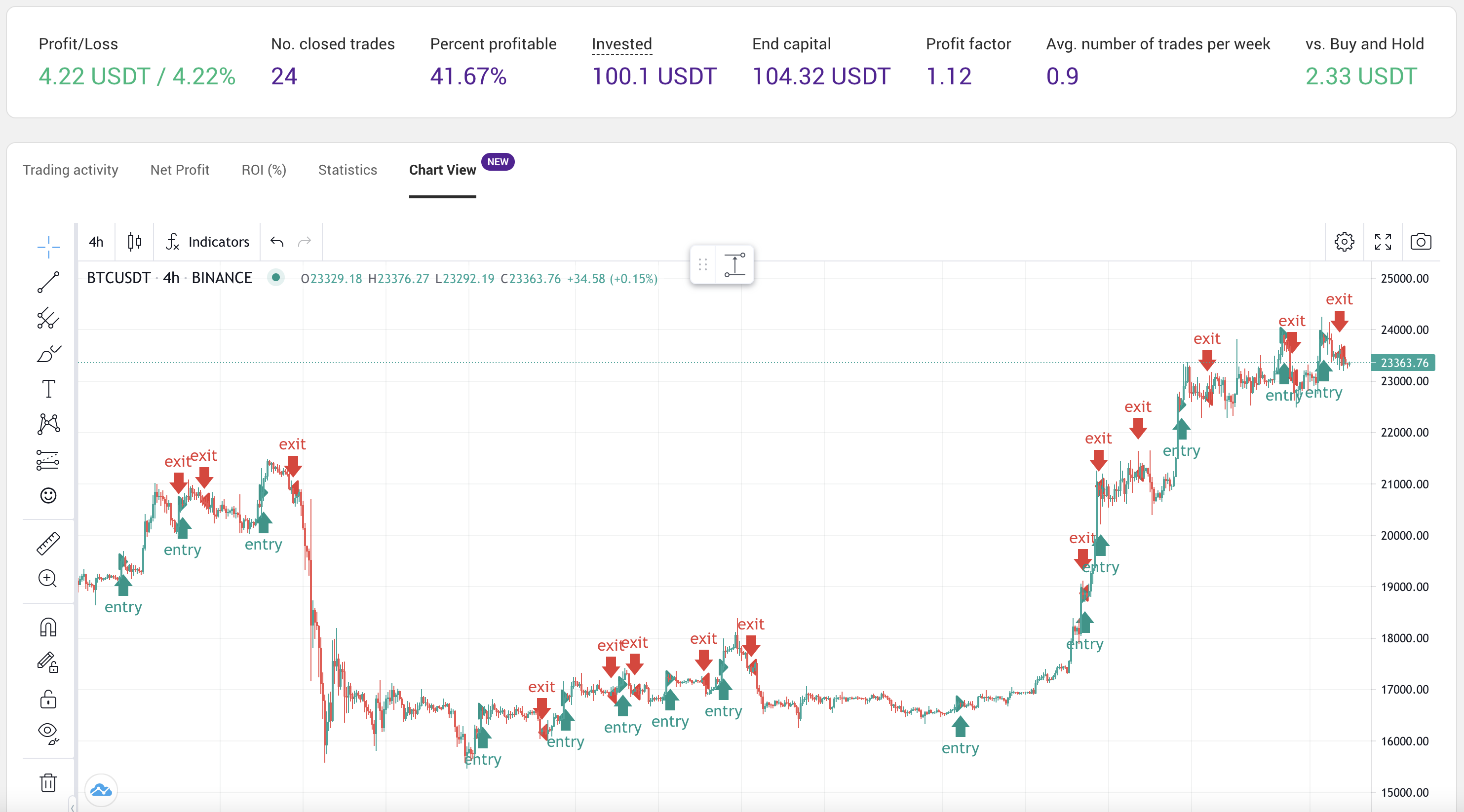# Simple MACD, EMA and Supertrend trading strategy template

## Strategy template description

This strategy template is a good starting point for a day trader or swing trader. It can be used on multiple timeframes (from 1 minute to 4 hours) and is a good addition to manual trades if you want to automate part of the work. The template is set to work on a 4h timeframe by default.

## Step 1: Login and click on Create Strategy

Once you're logged in to the Vestinda app, click on Create Strategy from the left menu.## Step 2: Use a strategy Template

Click on Use a Strategy Template from the overlay.

### Find the template in the list and click on "Use this Template" button.

Template name inside Vestinda app is  Template - MACD EMA Suppertrend. You can find it in the Templates page from here: https://app.vestinda.com/templates

✅ Everyone has access to templates. You can use it to create trading strategies without coding skills and jump-start learning.

## Step 3: Learn how the strategy works

Once you reach the template page, you have the entire strategy code ready to be used.

You can see the parameters for each indicator, but also modify the timeframe and all parameters.## Step 4: Backtest strategy or Demo Trade

Before any execution, backtesting the strategy is the best place to start from. You can do it on thousands of assets and many years of historical data.

This is helping you learn how to use these indicators, in what market conditions they work best, and what assets to trade using this strategy and indicators. Read more about Backtesting.

Demo Trading is another way to simulate the strategy without risking real money. This allows you to start using the strategy on the market moving forward on any available asset. Read more about Demo Trading.## Step 5: Results

This is the result of backtesting this template on BTCUSDT on a 6 months period from Aug 2022 to Feb 2023.

With a simple strategy like this, we got an outcome that returned better than the benchmark. Profitability is not great, but adding more filters to the entries can helpOnce you're confident enough, you can start Real Trading.

## Indicators in the strategy

#### MACD (Moving Average Convergence Divergence)

It is a trend-following momentum indicator that shows the relationship between two moving averages of a security’s price.

MACD stands for Moving Average Convergence Divergence and is a popular technical indicator used in stock trading and analysis. It is formed by plotting two lines on a price chart, the MACD line, and the signal line.

The MACD line is calculated by subtracting the 26-day exponential moving average (EMA) from the 12-day EMA of a given asset's price. The signal line is a 9-day EMA of the MACD line.

The MACD contains several elements that can be used to determine the momentum and trend of an asset's price, including:

1. Crossovers - When the MACD line crosses above the signal line, it is considered a bullish signal and may indicate a buying opportunity. Conversely, when the MACD line crosses below the signal line, it is considered a bearish signal and may indicate a selling opportunity.
2. Divergences - When the MACD line diverges from the price of an asset, it can indicate a potential reversal in the direction of the trend.
3. Histogram - The histogram represents the difference between the MACD line and the signal line and can be used to quickly visualize momentum and trend changes.

#### EMA (Exponential Moving Average)

Exponential Moving Average (EMA) is a type of moving average that places a higher weight on the more recent data points and is used to identify trends in an asset's price. Unlike a simple moving average, which gives equal weight to all data points in the calculation, an EMA gives more importance to the most recent prices, making it more responsive to recent price changes.

The EMA calculation involves smoothing out the fluctuations in the data by multiplying each data point by a weighting factor and adding the results together. The weighting factor is calculated based on the number of periods in the calculation and the time elapsed since each data point.

The EMA is often used in conjunction with other technical indicators to confirm trends, generate signals for buying or selling, and identify potential reversals in an asset's price. It can be used on any type of financial instrument, including stocks, currencies, commodities, and indices.

In summary, the EMA is a type of moving average that gives more weight to recent data points, making it a useful tool for identifying trends and potential price reversals in financial markets.

#### Supertrend

Supertrend is a technical indicator used in stock trading and analysis to identify trends in an asset's price. It is based on a combination of moving averages and the average true range, a measure of volatility.

The Supertrend indicator is plotted as a line on a price chart and is calculated using the following steps:

1. Calculation of ATR (Average True Range): The ATR is calculated using a moving average of the true range, which is the difference between the high and low prices of an asset for a given period.
2. Calculation of the basic Supertrend line: The Supertrend line is calculated by adding the ATR to the average of the closing price and the high price, or subtracting the ATR from the average of the closing price and the low price, depending on the trend of the asset's price.
3. Final Supertrend calculation: The final Supertrend is calculated by multiplying the basic Supertrend line by a factor that is based on the trend of the asset's price.

The Supertrend indicator can be used in two ways: as a trend-following indicator, to identify potential trend reversals, and to generate buy or sell signals.

## Congrats! 🎉

This template is inspired by the following video: